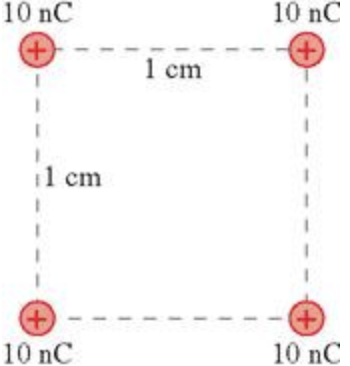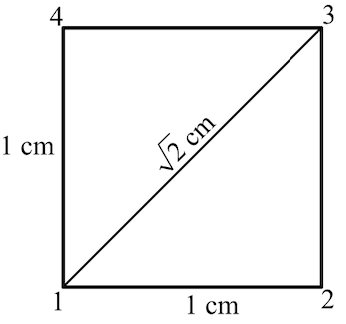In: Physics

The four 1.0 g spheres shown in the figure are released simultaneously and allowed to move away from each other.

The four 1.0 g spheres shown in the figure are released simultaneously and allowed to move away from each other.What is the speed of each sphere when they are very far apart?

Solutions

Expert Solution

Concepts and reason

The main concept used to solve the problem is electrostatic potential energy and kinetic energy. Initially, calculate total electrostatic potential energy of the system by calculating electrostatic potential energy between each pair. Later, when spheres are released, they start accelerating due to repulsive electrostatic force between them and this electrostatic potential energy starts getting converted into kinetic energy. Finally, When the separation between them gets sufficiently large, electrostatic energy becomes negligible as it gets totally converted into kinetic energy of the spheres.

Fundamentals

Electrostatic energy between two-point charges separated by a distance ' $$r$$ ' is defined as the amount of work done in bringing together charges initially separated by infinite distance to the final separation distance $$^{\prime} r$$ :

If $$Q 1$$ and $$Q 2$$ are two charges separated by a distance $$^{\prime} r$$; then electrostatic energy U between them is:

$$U=\frac{Q_{1} Q_{2}}{4 \pi \varepsilon_{0} r}$$

Here $$\varepsilon_{0}$$ is the permittivity of free space. $$\varepsilon_{0}=8.854187817 \ldots \times 10^{-12} F \cdot m^{-1}$$

The law of conservation of energy states that the energy of an isolated system remains conserved unless acted upon by some external force. Energy can neither be created, nor be destroyed, but can be transformed from one form to another. Here, in this case, it transforms from electrostatic potential energy to kinetic energy.

This figure shows the location of the charge.List all the charges and the distance between each pair. We have,

\begin{aligned} Q_{1} &=Q_{2} \\ &=Q_{3} \\ &=Q_{4} \\ &=10 \mathrm{nC} \end{aligned}

$$=10 \mathrm{nC}$$

Convert $$\mathrm{n} \mathrm{C}$$ to coulombs.

$$Q_{1}=(10 \mathrm{nC})\left(\frac{10^{-9} \mathrm{C}}{1 \mathrm{nC}}\right)$$

\begin{aligned}=& 10 \times 10^{-9} \mathrm{C} \\ &=10^{-8} \mathrm{C} \end{aligned}

And distance is,

\begin{aligned} r_{12} &=r_{23} \\ &=r_{34} \\ &=r_{14} \\ &=1 \mathrm{~cm} \end{aligned}

Convert from centimeter to meter.

$$r_{12}=(1 \mathrm{~cm})\left(\frac{10^{-2} \mathrm{~m}}{1 \mathrm{~cm}}\right)$$

$$=10^{-2} \mathrm{~m}$$

The diagonal distance is,

$$r_{13}=r_{24}$$

$$=\sqrt{1^{2}+1^{2}}$$

$$=(\sqrt{2} \mathrm{~cm})\left(\frac{10^{-2} \mathrm{~m}}{1 \mathrm{~cm}}\right)$$

$$=1.41 \times 10^{-2} \mathrm{~m}$$

The mass of the charge is,

\begin{aligned} m_{1} &=m_{2} \\ &=m_{3} \\ &=m_{4} \\ &=1 \mathrm{~g} \end{aligned}

Convert gram to kilogram.

\begin{aligned} m_{1}=&(1 \mathrm{~g})\left(\frac{10^{-3} \mathrm{~kg}}{1 \mathrm{~g}}\right) \\ &=10^{-3} \mathrm{~kg} \end{aligned}

The pairs with same value of charges and separation distance will have same value for potential energy associated with them.

Calculate the potential energy of the system. $$U_{12}=\frac{Q 1 Q 2}{4 \pi \varepsilon_{0} r_{12}}$$

Here, $$Q_{1}$$ and $$Q_{2}$$ are charge, $$\varepsilon_{0}$$ is permittivity of free space, and $$r_{12}$$ is distance between charge. Substitute, $$10^{-8} \mathrm{C}$$ for $$Q_{1}$$ and $$Q_{2}, 8.85 \times 10^{-12} \mathrm{~F} / \mathrm{m}$$ for $$\varepsilon_{0},$$ and $$10^{-2} \mathrm{~m}$$ for $$r_{12}$$

$$\begin{array}{c} U_{12}=\frac{\left(10^{-8} \mathrm{C}\right)\left(10^{-8} \mathrm{C}\right)}{4 \pi\left(8.85 \times 10^{-12} \mathrm{~F} / \mathrm{m}\right)\left(10^{-2} \mathrm{~m}\right)} \mathrm{J} \\ =9 \times 10^{-5} \mathrm{~J} \end{array}$$

Similarly,

$$U_{23}=U_{34}=U_{14}=9 \times 10^{-5} \mathrm{~J}$$

Now calculate potential energy between 1 and 3 . $$U_{13}=\frac{Q 1 Q 3}{4 \pi \varepsilon_{0} r_{13}}$$

Here, $$Q_{1}$$ and $$Q_{3}$$ are charge, $$r_{13}$$ is distance between them. Substitute, $$10^{-8} \mathrm{C}$$ for $$Q_{1}$$ and $$Q_{2}, 8.85 \times 10^{-12} \mathrm{~F} / \mathrm{m}$$ for $$\varepsilon_{0},$$ and $$1.41 \times 10^{-2} \mathrm{~m}$$ for $$r_{12}$$

$$\begin{array}{c} U_{13}=\frac{\left(10^{-8} \mathrm{C}\right)\left(10^{-8} \mathrm{C}\right)}{4 \pi\left(8.85 \times 10^{-12} \mathrm{~F} / \mathrm{m}\right)\left(1.41 \times 10^{-2} \mathrm{~m}\right)} \\ =6.38 \times 10^{-5} \mathrm{~J} \end{array}$$

And,

$$U_{24}=U_{13}$$

$$=6.38 \times 10^{-5} \mathrm{~J}$$

Now calculate the total energy of the system, $$U_{t o t}=U_{12}+U_{23}+U_{34}+U_{41}+U_{13}+U_{24}$$

Substitute $$9 \times 10^{-5} \mathrm{~J}$$ for $$U_{12}, U_{23}, U_{34}$$ and $$U_{41},$$ and $$6.38 \times 10^{-5} \mathrm{~J}$$ for $$U_{13}$$ and $$U_{24}$$

$$\begin{array}{c} U_{\mathrm{tot}}=\left(9 \times 10^{-5} \mathrm{~J}\right)+\left(9 \times 10^{-5} \mathrm{~J}\right)+\left(9 \times 10^{-5} \mathrm{~J}\right)+\left(9 \times 10^{-5} \mathrm{~J}\right)+\left(6.38 \times 10^{-5} \mathrm{~J}\right)+\left(6.38 \times 10^{-5} \mathrm{~J}\right) \\ =4.88 \times 10^{-4} \mathrm{~J} \end{array}$$

Electrostatic potential energy depends only upon magnitude of charges and distance between them. Hence, pairs with same value of charge and distance would have same amount of electrostatic potential energy associated with them. Electrostatic energy of each pair is added to get the total electrostatic energy of the system.

Consider the final velocity of each charge be $$v$$. Kinetic Energy, $$K . E=\frac{1}{2} m v^{2}$$

Total Kinetic energy of system,

$$\begin{array}{c} K . E_{t o t}=4\left(\frac{1}{2} m v^{2}\right) \\ =2 m v^{2} \end{array}$$

From the law of conservation of energy, $$K . E_{t o t}=U_{t o t}$$

Then, $$2 m v^{2}=U_{t o t}$$

Rearrange the equation $$2 m v^{2}=U_{\text {tot}}$$ for $$v$$. $$\boldsymbol{v}=\sqrt{\frac{U_{\mathrm{tot}}}{2 m}}$$

Substitute $$4.88 \times 10^{-4} \mathrm{~J}$$ for $$U_{\mathrm{tot}}, 10^{-3} \mathrm{~kg}$$ for $$m$$

$$v=\sqrt{\frac{4.88 \times 10^{-4} \mathrm{~J}}{2\left(10^{-3} \mathrm{~kg}\right)}}$$

$$=0.494 \mathrm{~m} / \mathrm{s}$$

The velocity of each sphere when they get very far apart from each other is $$0.49 \mathrm{~m} / \mathrm{s}$$.

since, all 4 spheres are identical in charge, mass and position, the force experienced by any sphere due to rest 3 is same for each sphere. Hence, the acceleration or rate of change of velocity would be same for each of them.1

Related Solutions

The figure shows a 100-kg block being released from rest from a height of 1.0 m.
The figure shows a 100-kg block being released from rest from a height of 1.0 m. It then takes it 0.90 s to reach the floor. What is the mass of the other block? The pulley has no appreciable mass or friction. A. 60 kg B. 48 kg C. 54 kg D. 42 kg             The figure shows a 100 - kg block being released from rest from a height of 1.0 m. It then takes it 0.90 s to reach...
The three masses shown in (Figure 1) are connected by massless, rigid rods. Assume that m1 = 180 g and m2 = 350 g.
The three masses shown in (Figure 1) are connected by massless, rigid rods. Assume that m1 = 180 g and m2 = 350 g. What is the x-coordinate of the center of mass? Express your answer with the appropriate units. What is the y-coordinate of the center of mass? Express your answer with the appropriate units.
Water flows steadily from an open tank as shown in the figure
Water Flowing from a Tank Water flows steadily from an open tank as shown in the figure. (Figure 1) The elevation of point 1 is $$10.0 \mathrm{~m},$$ anc the elevation of points 2 and 3 is $$2.00 \mathrm{~m}$$. The crosssectional area at point 2 is $$4.80 \times 10^{-2} \mathrm{~m}^{2} ;$$ at point 3 where the water is discharged, it is $$1.60 \times 10^{-2} \mathrm{~m}^{2}$$. The cross-sectional area of the tank is very large compared with the cross-sectional area of the...
Label the four mutated DNA segments shown below according to the type of point mutation each represents.
Label the four mutated DNA segments shown below according to the type of point mutation each represents. Use the codon table above to determine how each mutation would affect the amino acid coding for each segment. Drag the labels to their appropriate locations to identify the type of point mutation shown.
A copper loop hangs from two strings, as shown in (Figure 1).
A copper loop hangs from two strings, as shown in (Figure 1). Part B In which direction (toward or away from the magnet) does the loop swing if the magnet is moving toward the loop? toward the magnet away from the magnet Figure The loop doesn't move Part C In which direction (toward or away from the magnet) does the loop swing if the magnet is moving away from the loop? toward the magnet away from the magnet The loop...
if f and g are the functions whose graphs are shown, let u(x) = f(x)g(x) and v(x) = f(x)/g(x).
if f and g are the functions whose graphs are shown, let u(x) = f(x)g(x) and v(x) = f(x)/g(x) (a) Find u'(1) (b) Find v'(5).
Let P(x) = F(x)G(x) and Q(x) = F(x)/G(x), where F and G are the functions whose graphs are shown.
Let P(x) = F(x)G(x) and Q(x) = F(x)/G(x), where F and G are the functions whose graphs are shown.(a) Find P ' (2)(b) Find Q ' (7)
What will be the final speed of an electron released from rest at the negative plate?
A proton is released from rest at the positive plate of a parallel-plate capacitor. It crosses the capacitor and reaches the negative plate with a speed of 45000 m/s. What will be the final speed of an electron released from rest at the negative plate? Express your answer to two significant figures and include the appropriate units V=_________
The current in the wire shown in (Figure 1) is increasing
The current in the wire shown in (Figure 1) is increasing. Part A What is the direction of the induced current in loop A? clockwise counterclockwise No current is induced in this loop.   Part B What is the direction of the induced current in loop B? clockwise counterclockwise No current is induced in this loop.
Prepare journal entries to record each of the following four separate issuances of stock.
Prepare journal entries to record each of the following four separate issuances of stock. A corporation issued 5,000 shares of $5 par value common stock for$30,000 cash. A corporation issued 2,500 shares of no-par common stock to its promoters in exchange for their efforts, estimated to be worth $56,500. The stock has a$2 per share stated value. A corporation issued 2,500 shares of no-par common stock to its promoters in exchange for their efforts, estimated to be worth...